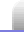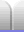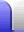# Mathematics

### Angle, Triangles & Pythagoras

• Angle Types – acute, right, obtuse, straight, reflex, complementary, and supplementary.

• Triangles – scalene, isoceles, equilateral, and right

• Triangle centres – incentre (and incentre), circumcentre (and circumcircle), centroid (and barycentre), orthocentre.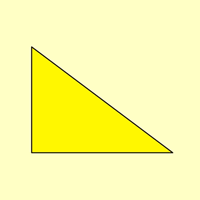### Length, Area and Volume

• Length – where pi comes from, and how to calculate the circumference of a circle.

• Area – you always wanted to know the surface area of a human, right?

• Volume – just remember that 1 liter is 1 cubic decimetre.

• Comparing Surface Area & Volume – the importance of surface area for volume, and how size makes a difference for chemical reactions and hot air balloons.

• Polygons & Polyhedra – polygons are shapes; polyhedra are solids. Objects such as soccer balls are made from certain polyhedrons, while dice can be made from many different polyhedra. Polyhedra can be made by plaiting; an example is given of a plaited cube.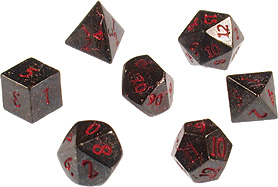• Measurement Devices – lots of devices used to measure all sorts of lengths, areas and volumes.

### Numbers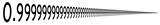• Prime Numbers – divisible only by themselves and 1.

• Complex Numbers – real and imaginary parts in one number. Square roots of negative numbers.

• Messing With Infinity – best left well alone.

• Prefixes – how measurement units are made easier to use with really big and really small numbers. This lists the 20 prefixes that are used by the Système International, plus an example of converting dimensions given in mm into a volume in litres.

### Fractions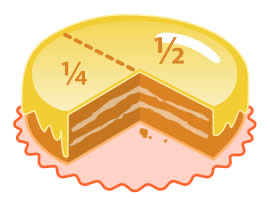### General Reference

• Mathematics Glossary – definitions of a few of the terms used in the maths section of this site. (A work in progress.)

• Measurement Devices – lots of devices used to measure everything from acceleration to voltage.

• Prefixes – the 20 prefixes that are used by the Système International.

• Time – to help in learning to tell the time there are some blank clock faces (PDF, 17KB) that can be downloaded and printed.

### Sequences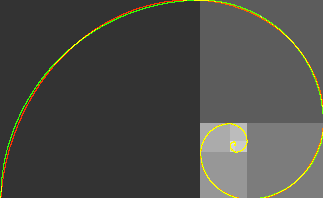### Expanding, Simplifying, Factorisation

Finding factors, and a few tricks for helping figure out if a number is divisible by some common factors.

### FunHome Astronomy Chemistry Electronics & Computers Mathematics Physics Field Trips Turn on javascript for email link